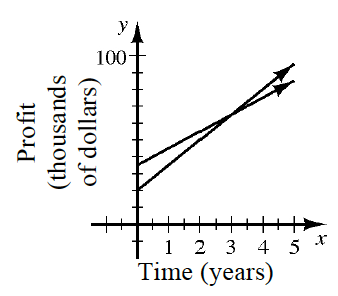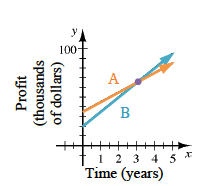### Home > AC > Chapter 2 > Lesson 2.1.5 > Problem2-54

2-54.The two lines at right represent the growing profits of Companies A and B. Homework Help ✎

1. Sketch this graph on your paper. If Company A started out with more profit than Company B, determine which line represents A and which represents B. Label the lines appropriately.

Company A's line should have a greater $y$-intercept, since they started with more profit than Company B.

See the graph below.2. In how many years will both companies have the same profit?

Find where the two lines intersect.

$3$ years

Determine the x-coordinate for this point.

3. Approximately what will that profit be?

The profit is the y-coordinate of the intercept. Each tick mark represents $10,000$.

4. Which company’s profits are growing more quickly? How can you tell?

Growth is related to how steep a line is. Which line is steeper?